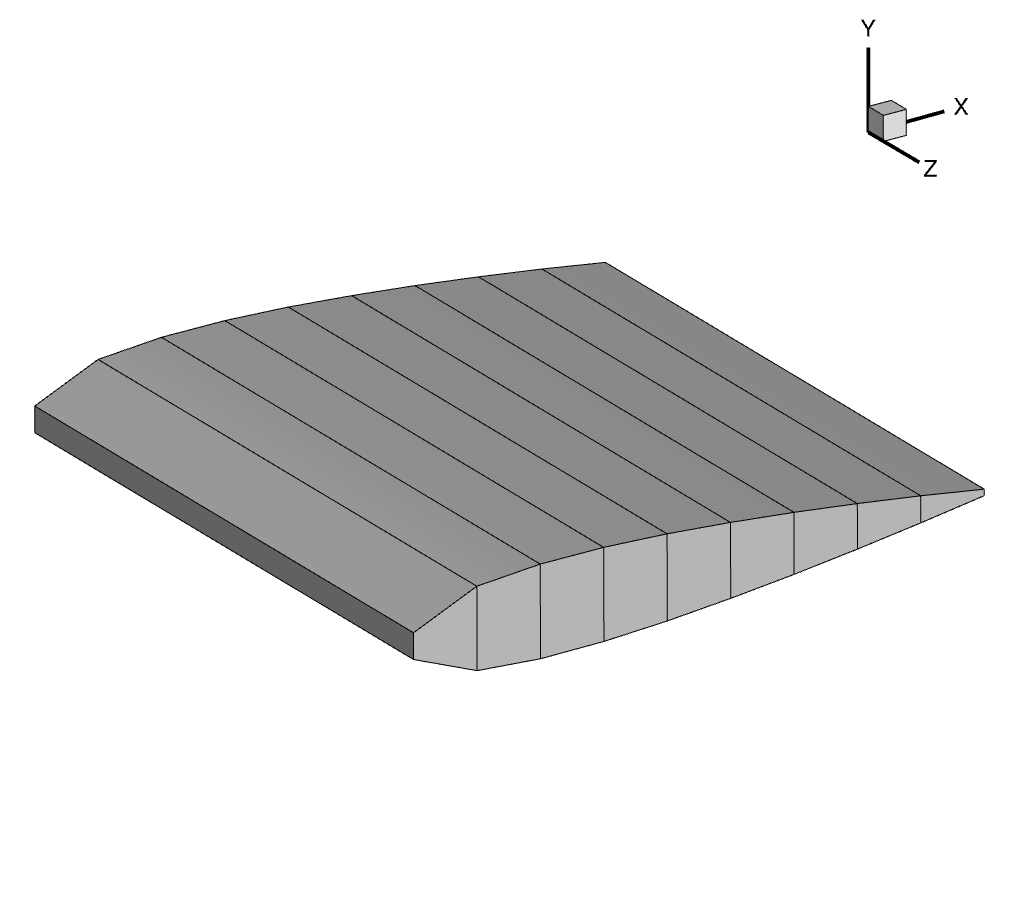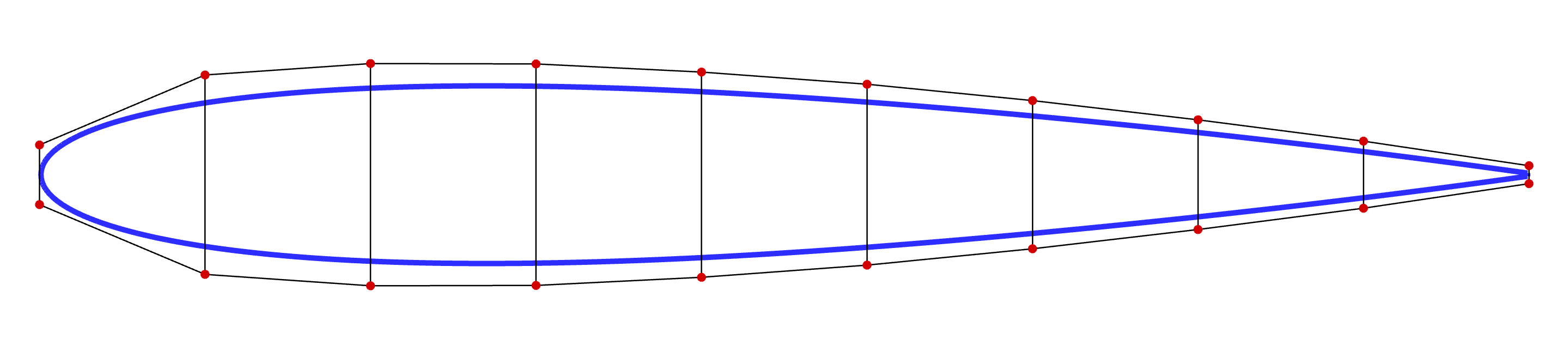# Geometric Parametrization

Next, the FFD (Free-Form Deformation) file has to be generated in PLOT3D format. This file contains the coordinates of the FFD points around the airfoil. These are control points that are fitted to the airfoil using B-splines, which are used to deform the airfoil.

The coordinates for the NACA0012 airfoil are in the file `n0012.dat`.

Navigate to the directory `airfoilopt/ffd` in your tutorial folder. Copy the airfoil data from `airfoilopt/mesh`:

```
span.prompt1:before {
content: "\$ ";
}
cp ../mesh/n0012.dat .
```

Create the following empty runscript in the current directory.

• `genFFD.py`

## Import Packages

```import numpy as np

```

```airfoil = np.loadtxt("n0012.dat")
npts = airfoil.shape
nmid = (npts + 1) // 2

```

The following two functions are used to get the upper and lower points of the airfoil.

```def getupper(xtemp):
myairfoil = np.ones(npts)
for i in range(nmid):
myairfoil[i] = abs(airfoil[i, 0] - xtemp)
myi = np.argmin(myairfoil)
return airfoil[myi, 1]

def getlower(xtemp):
myairfoil = np.ones(npts)
for i in range(nmid, npts):
myairfoil[i] = abs(airfoil[i, 0] - xtemp)
myi = np.argmin(myairfoil)
return airfoil[myi, 1]

```

## FFD Box Creation

The FFD box can now be set up.

```nffd = 10

FFDbox = np.zeros((nffd, 2, 2, 3))

xslice = np.zeros(nffd)
yupper = np.zeros(nffd)
ylower = np.zeros(nffd)

xmargin = 0.001
ymargin1 = 0.02
ymargin2 = 0.005

for i in range(nffd):
xtemp = i * 1.0 / (nffd - 1.0)
xslice[i] = -1.0 * xmargin + (1 + 2.0 * xmargin) * xtemp
ymargin = ymargin1 + (ymargin2 - ymargin1) * xslice[i]
yupper[i] = getupper(xslice[i]) + ymargin
ylower[i] = getlower(xslice[i]) - ymargin

```

`nffd` signifies the number of chordwise slices. we pre-allocate an array of generic size (a,b,c,3) to set up an empty FFD box. In this example, a=nffd (number of chordwise sections), b=c=2 (number of spanwise and thickness-wise sections respectively) and the final 3 is “fixed” as we are using 3D coordinates for each point

An empty FFD box is created.

`xmargin` and `ymargin` specify the closest distance from the airfoil to place the FFD box. `xslice`, `yupper`, and `ylower` store the x- and y- coordinates of the control points for each slice along the chord, taking into account the margins from the airfoil.

```# X
FFDbox[:, 0, 0, 0] = xslice[:].copy()
FFDbox[:, 1, 0, 0] = xslice[:].copy()
# Y
# lower
FFDbox[:, 0, 0, 1] = ylower[:].copy()
# upper
FFDbox[:, 1, 0, 1] = yupper[:].copy()
# copy
FFDbox[:, :, 1, :] = FFDbox[:, :, 0, :].copy()
# Z
FFDbox[:, :, 0, 2] = 0.0
# Z
FFDbox[:, :, 1, 2] = 1.0

```

The x- and y- coordinates are transferred to the `FFDbox` variable. Since the airfoil slices are the same along the z-direction, the x- and y- coordinates are copied over. The z-coordinates are updated to 0 and 1.

## Writing to File

```with open("ffd.xyz", "w") as f:
f.write("1\n")
f.write(str(nffd) + " 2 2\n")
for ell in range(3):
for k in range(2):
for j in range(2):
for i in range(nffd):
f.write("%.15f " % (FFDbox[i, j, k, ell]))
f.write("\n")
```

## Run it yourself!

You can now run the python file with the command:

```python genFFD.py
```

The above script writes the FFD coordinates to a PLOT3D `.xyz` file, which will be used for optimization.3D view of the FFD volumes2D view of the FFD volume, together with the embedded airfoil